# Google Code Jam Archive — Round 1A 2009 problems

## Overview

Round 1A was the first round of Code Jam 2009 in which contestants competed simultaneously; and what a job they did! 2499 contestants submitted at least one attempt, using a total of 25 different languages and solving a total of 3543 inputs.

Problems A-large, B and C were all tough, and solving A-small alone in the first 45 minutes was enough to allow several contestants to advance. Only 64 contestants successfully solved every problem in this tough set. Congratulations to them, and to the top 1000, who advanced to Round 2!

Cast

Problem A. Multi-base happiness Written by Tyler Neylon. Prepared by Xiaomin Chen and Daniel Rocha.

Problem B. Crossing the Road Written by Mohamed Eldawy. Prepared by Bartholomew Furrow.

Problem C. Collecting Cards Written by Derek Kisman. Prepared by Frank Chu.

Contest analysis presented by Xiaomin Chen, Bartholomew Furrow, and Pablo Dal Lago.

Solutions and other problem preparation provided by Marius Andrei, Tomek Czajka, Ante Derek, John Dethridge, Petr Mitrichev, Fábio Moreira, and Igor Naverniouk.

## A. Multi-base happiness

### Problem

Given an integer N, replace it by the sum of the squares of its digits. A happy number is a number where, if you apply this process repeatedly, it eventually results in the number 1. For example, if you start with 82:

```8*8 + 2*2       = 64 + 4    = 68,  repeat:
6*6 + 8*8       = 36 + 64   = 100, repeat:
1*1 + 0*0 + 0*0 = 1 + 0 + 0 = 1 (happy! :)
```

Since this process resulted in 1, 82 is a happy number.

Notice that a number might be happy in some bases, but not happy in others. For instance, the base 10 number 82 is not a happy number when written in base 3 (as 10001).

You are one of the world's top number detectives. Some of the bases got together (yes, they are organized!) and hired you for an important task: find out what's the smallest integer number that's greater than 1 and is happy in all the given bases.

### Input

The first line of input gives the number of cases T. T test cases follow. Each case consists of a single line. Each line contains a space separated list of distinct integers, representing the bases. The list of bases is always in increasing order.

### Output

For each test case, output:

`Case #X: K`
where X is the test case number, starting from 1, and K is the decimal representation of the smallest integer (greater than 1) which is happy in all of the given bases.

### Limits

Time limit: 60 seconds per test set.
Memory limit: 1 GB.
2 ≤ all possible input bases ≤ 10

### Small dataset

1 ≤ T ≤ 42

2 ≤ number of bases on each test case ≤ 3

### Large dataset

1 ≤ T ≤ 500

2 ≤ number of bases on each test case ≤ 9

### Sample

Sample Input
```3
2 3
2 3 7
9 10
```
Sample Output
```Case #1: 3
Case #2: 143
Case #3: 91
```

### Important Note

Please remember that you must submit all code used to solve the problem.

### Problem

Where roads intersect, there are often traffic lights that tell pedestrians (people walking) when they should cross the street. A clever pedestrian may try to optimize her path through a city based on when those lights turn green.

The city in this problem is a grid, N rows tall by M columns wide. Our pedestrian wants to get from the northeast corner of the southwest block to the southwest corner of the northeast block. Your objective is to help her find her way from corner to corner in the fastest way possible.

The pedestrian can cross a street in 1 minute, but only if the traffic light is green for the entire crossing. The pedestrian can move between two streets, along one edge of a block, in 2 minutes. The pedestrian can only move along the edges of the block; she cannot move diagonally from one corner of a block to the opposite corner.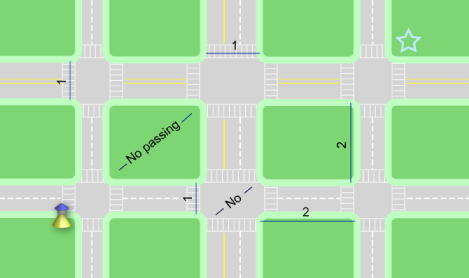Traffic lights follow the following pattern: at intersection i, the north-south lights stay green for Si minutes, while the east-west lights stay red. Then the north-south lights turn red, the east-west lights turn green, and they stay that way for Wi minutes. Then they start the same cycle again. The pedestrian starts moving at t=0 minutes; traffic light i starts a cycle by turning green in the north-south direction at t=Ti minutes. There are cycles before t=Ti as well.

For example, intersection 0 could have the following values:

``` S0 = 3, W0 = 2, T0 = 0 ```

The north-south direction turns green after 0 minutes. That lasts 3 minutes, during which time the pedestrian can cross in the north-south direction and not the east-west direction. Then the lights switch, and for the next 2 minutes the pedestrian can cross in the east-west direction and not the north-south direction. Then, 5 minutes after it started, the cycle starts again. This is exactly the same as the following configuration:

``` S0 = 3, W0 = 2, T0 = 10 ```

### Input

The first line in the input contains the number of test cases, C. This is followed by C test cases in the following format:

A single line containing "N M", where N and M are the number of horizontal roads (rows) and vertical roads (columns), as above. This is followed by N lines. The ith of those lines contains information about intersections on the ith row, where the 0th row is the northmost. Each of those lines will contain 3M integers, separated by spaces, in the following form:

``` Si,0 Wi,0 Ti,0 Si,1 Wi,1 Ti,1... Si,M-1 Wi,M-1 Ti,M-1 ```

Si,j, Wi,j and Ti,j all refer to the intersection in the ith row from the north and the jth column from the west.

### Output

For each test case, output a single line containing the text "Case #x: t", where x is the number of the test case and t is the minimum number of minutes it takes the pedestrian to get from the southwest corner to the northeast corner.

### Limits

Time limit: 30 seconds per test set.
Memory limit: 1 GB.

C, N, M, Si,j, Wi,j, Ti,j are all non-negative integers.
C ≤ 100

### Small Input

1 ≤ N, M ≤ 3
0 < Si,j, Wi,j ≤ 10
0 ≤ Ti,j ≤ 20

### Large Input

1 ≤ N, M ≤ 20
0 < Si,j, Wi,j ≤ 107
0 ≤ Ti,j ≤ 108

### Sample

Sample Input
```2
1 1
3 2 10
1 2
1 5 3 1 5 2
```
Sample Output
```Case #1: 4
Case #2: 7
```

### Explanation

The first case is described above. The pedestrian crosses to the North (1 minute), waits 2 minutes and then crosses to the East (1 minute), for a total of 4 minutes.

The second case is depicted in the diagram below. The pedestrian crosses to the East (1 minute), waits 2 minutes and crosses to the North (1 minute). Then she walks east a block (2 minutes) and crosses to the East (1 minute) for a total of 7 minutes.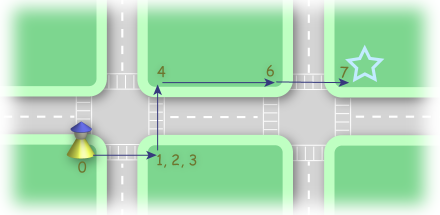## C. Collecting Cards

### Problem

You've become addicted to the latest craze in collectible card games, PokeCraft: The Gathering. You've mastered the rules! You've crafted balanced, offensive, and defensive decks! You argue the merits of various cards on Internet forums! You compete in tournaments! And now, as they just announced their huge new set of cards coming in the year 2010, you've decided you'd like to collect every last one of them! Fortunately, the one remaining sane part of your brain is wondering: how much will this cost?

There are C kinds of card in the coming set. The cards are going to be sold in "booster packs", each of which contains N cards of different kinds. There are many possible combinations for a booster pack where no card is repeated. When you pay for one pack, you will get any of the possible combinations with equal probability. You buy packs one by one, until you own all the C kinds. What is the expected (average) number of booster packs you will need to buy?

### Input

The first line of input gives the number of cases, T. T test cases follow, each consisting of a line containing C and N.

### Output

For each test case, output one line in the form

```Case #x: E
```
where x is the case number,starting from 1, and E is the expected number of booster packs you will need to buy. Any answer with a relative or absolute error at most 10-5 will be accepted.

### Limits

Time limit: 30 seconds per test set.
Memory limit: 1 GB.
1 ≤ T ≤ 100

1 ≤ NC ≤ 10

1 ≤ NC ≤ 40

### Sample

Sample Input
```2
2 1
3 2
```
Sample Output
```Case #1: 3.0000000
Case #2: 2.5000000
```

## Analysis — A. Multi-base happiness

How can you tell if a number is unhappy? You can tell if it enters a cycle when you apply the process described in the problem. Here is one example, in base 10:

```42 → 20 → 4 → 16 → 37 → 58 → 89 → 145 → 42 repeated
```
Naturally, x → y denotes that y is the next number if we apply the process on x. Starting from any number, if you apply the process, eventually you will reach 1 or enter one of those cycles.

But is that really true? A careful reader might ask: Couldn't the process keep hitting new numbers, and never enter a cycle? Look at the cycle for 42: Before you hit 42 again, the numbers just jump around without a clear pattern.

It turns out that this can never happen: that there are only finitely many such cycles, and they're all finite in length. In fact, in base 10, this is the only one! All the numbers involved in such a cycle must be reasonably small. Indeed, when you start a number that is big (think about 99999..9999), applying the process will lead to numbers that become smaller and smaller very rapidly. One can easily prove that, in any base B, there is a threshold H of O(B3) such that any number larger than H will have a smaller successor.

Another question is: Given a set of bases, does there exist a number that is happy in all of them? We don't know the answer to that question, but based on our computation we know such numbers exist for all bases up to 10. On the other hand, if you intuit that the property of a number being happy is somehow random, and somehow independent across different bases, then you can believe that multi-base happiness is rare, and that the density of such numbers decreases exponentially with the number of bases. In our problem. The smallest happy number for the input (2, 3, 4, 5, 6, 7, 8, 9, 10) is 11814485; just barely affordable with a brute-force search.

In the computation, one obvious trick is to cache, for a pair (x, B), whether x is happy in base B, so we can avoid following the jumping sequence or the cycles every time. We only need to do this for small values of x -- for example all x ≤ 1000 would be more than enough -- since any 32-bit integer bigger than 10000 becomes smaller than 1000 in one step.
Since the maximum base is 10, one may realize that there are only 29 - 10 = 502 possible distinct inputs. So why don't we just calculate them all? This can actually be faster than solving the input cases one by one, if we solve the smaller sets first. For a set S of bases, we do not need to start the search from 2; we can start from the answer for any S' ⊂ S, since a number happy in all bases from S is at least happy in all bases from S'.

If you think your implementation is still not fast enough. Then run your program while you are solving other problems. There are only 502 possible input cases. Solve all of them, produce the list of answers, and then start the submission process; just don't forget you also need to submit the slow program that produced the list. This is why we had a special note at the bottom of the problem statement.

Wikipedia article: Happy Numbers

Test Data
info We recommend that you practice debugging solutions without looking at the test data.

## Analysis — B. Crossing the Road

A grid of roads; a person moving from place to place; this problem has all the hallmarks of a graph problem, and a shortest path problem at that. Our pedestrian is trying to get from one corner of the map to the opposite corner. Some steps that she takes can be made at any time, and take 2 minutes; for others, she has to wait until the light turns green, and then take another minute. How can we minimize the time she takes to reach her goal?

To solve this problem, we need to describe the state of the world. The pedestrian can be in any of 2m positions in the x direction, in any of 2n positions in the y direction, and at some time t. Since the pedestrian can always just wait if she needs to, she would always prefer to be at (x, y, t) over (x, y, t+1); so an algorithm for computing the earliest time at which the pedestrian can arrive at (x, y) will solve this problem.

This problem differs from a standard graph problem in that, for two neighboring locations across a road from one another, the weight of the edge between them is not fixed. As it turns out this is only a minor complication, and we can use slightly modified versions of some standard shortest-path algorithms.

From each location, the pedestrian can go north, south, east or west, unless the direction in question is off the edge of the map. The amount of time this takes will be 2 minutes if it's along a block, or (the amount of time until the traffic light is green) + 1 minute if it's to cross a road.

Bellman-Ford is probably the easiest algorithm to implement for this. The longest path will take at most O(m+n) steps, so your algorithm will terminate in at most that many stages. At each stage, for each point, you'll try to update its (up to) four neighbors. The running time is O(nm(n+m)).

Dijkstra's algorithm is usually more efficient. With 4nm states and 4 directions in which the pedestrian can move from each state, this algorithm will complete in O(nm log(nm)) time.

The correctness of either algorithm can be proved in almost the same way as its standard counterpart. We leave these proofs as worthy exercises for the reader.

Test Data
info We recommend that you practice debugging solutions without looking at the test data.

## Analysis — C. Collecting Cards

This problems requires some basic knowledge of probability and combinatorics. We want to calculate the expected number of packs we need to buy to obtain all C different cards.

Let's denote by E(x) the expected number of packs we would need to buy if we started with x different cards (it doesn't matter what those cards are). The answer to the problem is the value for E(0). We also know that E(C) = 0, because if we already have C different cards we don't need to buy any additional packs.

We can derive useful equations for other values of E(x) by thinking about all the possible outcomes after buying one additional pack. Let's call T(x,y) the probability of ending up with y different cards after opening a new pack. Then we have the following equation for E(x):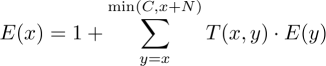We need to buy at least one new pack, so that's where the 1 comes from. The expected number of packs we need to buy after that depends on how many new cards we get. If we end up with y different cards we need to add the expected number of packs to reach C starting from y, which we called E(y), multiplied by the probability of this particular alternative given by T(x, y).

All these equations put together form a system of linear equations with an upper triangular matrix which can be solved using the standard back substitution method.

But we still haven't said how to calculate the entries of the matrix T (that is, the values of T(x, y) for all different x and y). We'll calculate this with the help of binomial coefficients: the number of different possible packs is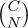. To end up with y different cards, we need to choose y-x out of C-x possible new cards, and the remaining N-(y-x) have to be chosen from the x cards we already have. The answer then is: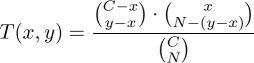(For those with some knowledge of probability, this is called the hypergeometric distribution).

The special case, where N = 1, is the well known coupon collector's problem.

Test Data
info We recommend that you practice debugging solutions without looking at the test data.

## Statistics — B. Crossing the Road

### Test set 1: 213 correct solutions (10.6% solve rate)

First
CS.Ferng C++, 42:54
rem C++, 43:18
TripleM C++, 44:15
Eryx C++, 44:36
Jimb C++, 45:13
Shortest
tharkang Python, 1427 bytes
fsouza C++, 1452 bytes
zhuojie aka Zhuojie C++, 1515 bytes
gagguy C++, 1581 bytes
ricardohahn aka RicardoHahn C++, 1622 bytes

### Test set 2: 172 correct solutions (8.6% solve rate)

First
rem C++, 44:14
CS.Ferng C++, 44:57
Eryx C++, 45:38
TripleM C++, 49:58
dolphinigle aka Dolph C++, 56:25
Shortest
tharkang Python, 1427 bytes
gagguy C++, 1445 bytes
zhuojie aka Zhuojie C++, 1515 bytes
ricardohahn aka RicardoHahn C++, 1706 bytes
Farmer.John C++, 1758 bytes